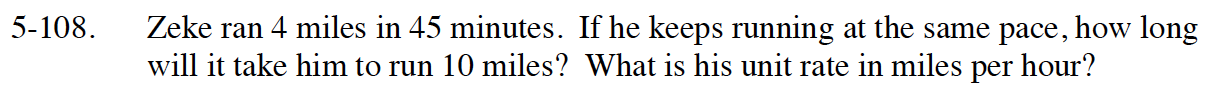Home > INT1 > Chapter Ch5 > Lesson 5.3.2 > Problem5-108

5-108.

Zeke ran 4 miles in 45 minutes. If he keeps running at the same pace, how long will it take him to run 10 miles? What is his unit rate in miles per hour? Homework Help ✎A proportion can be used to solve this.

$\frac{\text{4 miles}}{\text{45 minutes}}=\frac{\text{10 miles}}{x\text{ minutes}}$

${\text{To find the unit rate, consider another proportion where one ratio is }\frac{\textit{x} \text{ miles}}{\text{60 minutes}}}$